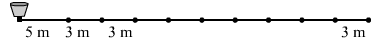# In a potato race, a bucket is placed at the starting point, which is 5 m from the first potato, and the other potatoes are placed 3 m apart in a straight line.

Question:

In a potato race, a bucket is placed at the starting point, which is 5 m from the first potato, and the other potatoes are placed 3 m apart in a straight line. There are 10 potatoes in the line. A competitor starts from the bucket, picks up the nearest potato, runs back with it, drops it in the bucket, runs back to pick up the next potato, runs to the bucket to drop it in, and he continues in the same way until all the potatoes are in the bucket. What is the total distance the competitor has to run?Solution:

Distance covered by the competitor to pick and drop the first potato = 2 × 5 m = 10 m

Distance covered by the competitor to pick and drop the second potato = 2 × (5 + 3) m = 2 × 8 m = 16 m

Distance covered by the competitor to pick and drop the third potato = 2 × (5 + 3 + 3) m = 2 × 11 m = 22 m and so on.

∴ Total distance covered by the competitor = 10 m + 16 m + 22 m + ... up to 10 terms

This is an arithmetic series.

Here, = 10, d = 16 − 10 = 6 and n = 10

Using the formula, $S_{n}=\frac{n}{2}[2 a+(n-1) d]$, we get

$S_{10}=\frac{10}{2}[2 \times 10+(10-1) \times 6]$

$=5 \times(20+54)$

$=5 \times 74$

$=370$

Hence, the total distance the competitor has to run is 370 m.